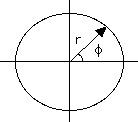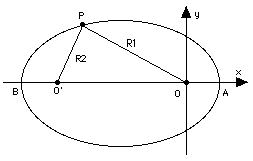# Disclaimer: The following material is being kept online for archival purposes.

## Although accurate at the time of publication, it is no longer being updated. The page may contain broken links or outdated information, and parts may not function in current web browsers.

 Site Map Lesson plan Glossary Timeline Questions & Answers Central Home Page

# (11a)  Ellipses and Kepler's First LawIndex9b. The Planets9c. Copernicus
to Galileo10. Kepler's Laws

Kepler's Laws
(For teachers)10a. Scale of Solar Sys.11. Graphs & Ellipses11a. Ellipses
and First Law12. Second Law12a. More on 2nd Law12b. Orbital Motion12c. Venus transit (1)12d. Venus transit (2)12e. Venus transit (3)

Newtonian Mechanics13. Free Fall

As already noted, other ways exist for labeling points in the plane. For instance, a point P may be labeled by its distance r from a central point O ("origin") and the angle φ (or Greek f, also written φ) which the line OP makes with some standard direction. Such "polar coordinates" (drawing on the left, below) are the ones best suited for describing planetary motion.

## The Ellipse in Polar Coordinates

Again, if all the values of (r, φ) of a curve are related by some equation which can be symbolically written

r = r(φ)

then the function r(f) is said to be the equation of the line, in polar coordinates. The simplest function is a constant number a, giving the line

r = aThe value of r equals a for any value of φ. That gives a circle around the origin, its radius equal to a, shown in the drawing on the right above.

## The Ellipse

Consider next the curve whose equation is

r   =   a(1– e2) / (1+ e cos φ)

where the eccentricity e is a number between 0 and 1. If e = 0, this is clearly the circle encountered earlier.

How about other values? The function cos φ represents a wave-like behavior (picture below), and as φ goes through a full circle, it goes down, from +1 to 0, then –1, then up again to 0 and +1. The denominator also rises and falls as a wave, and it is smallest when cos φ = –1 . Here is the table of the main values (360 is in parentheses, because it represents the same direction as 0 degrees):

As already noted, other ways exist for labeling points in the plane. For instance, a point P may be labeled by its distance r from a central point O ("origin") and the angle φ (Greek f) which the line OP makes with some standard direction. Such "polar coordinates" (drawing on the left, below) are the ones best suited for describing planetary motion.

## The Ellipse in Polar Coordinates

Again, if all the values of (r, φ) of a curve are related by some equation which can be symbolically written

r = r(φ)

then the function r(f) is said to be the equation of the line, in polar coordinates. The simplest function is a constant number a, giving the line

r = aThe value of r equals a for any value of φ. That gives a circle around the origin, its radius equal to a, shown in the drawing on the right above.

## The Ellipse

Consider next the curve whose equation is

r = a(1– e2)/(1+ e cos φ)

where the eccentricity e is a number between 0 and 1. If e = 0, this is clearly the circle encountered earlier.

How about other values? The function cos φ represents a wave-like behavior (picture below), and as φ goes through a full circle, it goes down, from +1 to 0, continues to –1, then up again to 0 and +1. The denominator also rises and falls as a wave, and it is smallest when cos φ = –1 . Here is the table of the main values (360 is in parentheses, because it represents the same direction as 0 degrees):φ degrees 0 90 180 270 (360) cos φ 1 0 –1 0 1 1 + e cos φ 1 + e 1 1 – e 1 1 + e
 As long as e is less than 1, the denominator is always positive. It is never zero, so that for any f one can name, one can always find a suitable r. In other words, the curve goes completely around the origin, it is closed.    The expression (1 – e2) can be factored--that is, written as two expressions multiplied by each other ("the product of two expressions"). As discussed in the section on algebraic identities 1 – e2 =  (1 – e)(1 + e) At some of the points on the above table, either (1 – e) or (1 + e) cancels the denominator, giving:
 φ degrees 0 90 180 270 (360) r a(1 – e) a(1 – e2) a(1 + e) a(1 – e2) a(1 – e)
 The distance of the line from the origin thus fluctuates between a(1 – e) and a(1 + e), and the result is a flattened circle or ellipse; the point O (the origin) is its focus. All planetary orbits resemble ellipses, each with its own value of e or eccentricity: the smaller e is, the closer the shape to a circle. The Earth's orbit is very close to a circle, with e = 0.0168, and other major planets (except for Pluto) have comparable eccentricities: if you saw a scale drawing of that orbit on a sheet of paper, your eye would not be able to tell it apart from a circle. The orbit of Comet Halley, on the other hand, has e quite close to 1.The collection of all points for   which R1 + R2 has the same value  is an ellipse

As mentioned in the preceding section a second focus O' can be drawn symmetrical to O, and the ellipse can be defined (its original definition, in fact) as the collection of points for which the sum R1+R2 of their distances from O and O' is always the same

The longest dimension of the ellipse, its width AB along the line connecting the two foci, is its "major axis." Suppose (R1,R2) are the distances of A from the foci O and O'. Then R1 = OA =a(1–e) is the smallest distance of the ellipse from O, R2 = O'A = OB (by symmetry) is the largest and therefore equals a(1 + e). But, OA + OB = AB, hence

 AB = a(1 – e) + a(1 + e) = 2a

Hence the quantity a in the equation of the ellipse is known as semi-major axis. We can now state Kepler's law more precisely as "the square of the orbital period T is proportional to the cube of the semi-major axis a of its orbit around the Sun."

The two quantities (a,e) completely define the ellipse. When that ellipse is the orbit of a planet or a satellite, they form two of the six orbital elements which define the state of the orbiting body. A third element, the mean anomaly M, specifies the position of the planet or satellite along the orbit, and the remaining three define the orientation of the orbit in 3-dimensional space. More about the orbital elements can be found in the optional section (12b)

## Refining the First Law

Originally, Kepler's first law was:

"The orbit of a planet is an ellipse, with the Sun at one focus"

Actually, this is not 100% accurate. Imagine the planet magically getting heavier and heavier, while the Sun is getting lighter and lighter. At some point both of them would be equally heavy: could we then say which is orbiting around which?

To be fully accurate, the first law should have placed the focus of the orbital ellipse at the center of gravity of the planet-Sun system. (The center of gravity will be defined later, but intuitively, if the masses are very unequal, as with a planet and the Sun, it lies close to the center of the heavier object.) Because the Sun is so much heavier than the Mars, the effect on the orbit of Mars (which Kepler studied) was too small to be noted by him. Nevertheless, the Sun also moves in response to motions of its planets, and motions of this type have become an important tool in the search for planets outside the solar system.

Recently a few such planets have been found, but most are Jupiter-sized and none seems suitable for life. The search, however, goes on. One discovery (15 April 1999, "recent" when this web site first appeared) has been the system of upsilon Andromeda which appears to contain at least 3 planets. For another recent discovery associated with a distant planet, click here.

Extra-solar planets are discussed in more detail in the next section, which duplicates a few of the paragraphs above.

Bodies in the solar system can also move in other conic sections, in parabolas or hyperbolas, whose equations resemble that of an ellipse, but have e equal to 1 or larger. Properly speaking, these orbits do not have a "semi-major axis," because they are not limited in size like ellipses but extend to infinity. Instead, we must write

r = p/(1 + e cos φ)

which doe not raise any problems at e=1. Such bodies are not bound to the Sun, but are free to escape it. The denominator in the equation of the trajectory then becomes zero for some values of φ, making its r infinite, and as the moving body approaches those values, it moves further and further away, with no limit. Comets in general have an eccentricity e close to 1, suggesting they have come from the very distant fringes of the solar system. The space probes Voyager 1 and 2 haves e > 1 and are on its way out of the solar system, never to return.

Questions from Users:   Asymmetry of the Moon's orbit
Also asked:   Shape of the orbit of Mars
Yet another:   The varying distance between Earth and Sun
***     Shape of the Earth's Orbit
***     Search for planets outside our solar system
***     Why are planetary orbits eccentric?

Author and Curator:   Dr. David P. Stern
Mail to Dr.Stern:   stargaze("at" symbol)phy6.org .

Originally posted 12 December 2004, last updated: 6 April 2014

# Above is background material for archival reference only.

NASA Official: Adam Szabo

Curators: Robert Candey, Alex Young, Tamara Kovalick